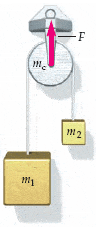# Acceleration of two masses on string

cowmoo32

## Homework Statement

In the Atwood's machine below the string passes over a fixed cylinder of mass mc. The cylinder does not rotate. Instead, the string slides on its frictionless surface. (Use any variable or symbol stated above along with the following as necessary: m1, m2, and g. Find the magnitude only.)F = ma

## The Attempt at a Solution

m1 ƩFY: T-m1g = m1a

T = m1(a + g)

m2 ƩFY: T - m2g = -m2a

Substitute T in

m1a + m1g - m2g = -m2a

a(m1 + m2) = g(m2 - m1)

a = g(m2 - m1) / (m1 + m2)

This seems simple enough, but it's not being counted as correct. The acceleration for the center of mass for the system would be the same as the acceleration of the individual blocks, correct?

## Answers and Replies

voko
If we are to take picture as an indication of the masses involved, then m1 > m2, and your acceleration is negative. You, however, have been asked for its magnitude only, which must not be negative. If we ignore the picture, then you must ensure you get non-negative magnitudes irrespective of what the masses are.

cowmoo32
I haven't been given any values and have to solve the problem symbolically. So ignoring the picture, is my math correct?

voko
The math is correct, but it is not finished. You need the magnitude of the acceleration that you have derived.

cowmoo32
voko
I have looked at the reference, and absolute value is covered. But perhaps you really just need to take the picture as a cue.

voko
Disregard that - I just re-read your latest message. You need to derive the motion of the center of mass. What is its equation?

cowmoo32
I tried absolute value and it's still incorrect. I'll have to read over my notes again because clearly something is missing.

typhowowl
So the acceleration of the center of mass is a_cm=(m_1*a-m_2*a)/(m_1+m_2+m_3) since m_1 and m_2 have opposite accelerations. Plug in a and you should arrive at the answer. (note that I assumed m_1 was heavier).